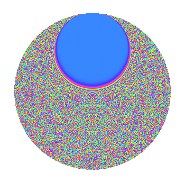# Properties

 Label 4.38.a.aLevel 4 Weight 38 Character orbit 4.a Self dual Yes Analytic conductor 34.686 Analytic rank 0 Dimension 3 CM No Inner twists 1

# Related objects

## Newspace parameters

 Level: $$N$$ = $$4 = 2^{2}$$ Weight: $$k$$ = $$38$$ Character orbit: $$[\chi]$$ = 4.a (trivial)

## Newform invariants

 Self dual: Yes Analytic conductor: $$34.6856152498$$ Analytic rank: $$0$$ Dimension: $$3$$ Coefficient field: $$\mathbb{Q}[x]/(x^{3} - \cdots)$$ Coefficient ring: $$\Z[a_1, \ldots, a_{5}]$$ Coefficient ring index: $$2^{24}\cdot 3^{5}\cdot 7$$ Fricke sign: $$-1$$ Sato-Tate group: $\mathrm{SU}(2)$

## $q$-expansion

Coefficients of the $$q$$-expansion are expressed in terms of a basis $$1,\beta_1,\beta_2$$ for the coefficient ring described below. We also show the integral $$q$$-expansion of the trace form.

 $$f(q)$$ $$=$$ $$q$$ $$+ ( -90721164 - \beta_{1} ) q^{3}$$ $$+ ( 1213681705398 + 1822 \beta_{1} + \beta_{2} ) q^{5}$$ $$+ ( 504728169053864 + 709926 \beta_{1} + 420 \beta_{2} ) q^{7}$$ $$+ ( 34317977529352221 - 24221340 \beta_{1} + 34830 \beta_{2} ) q^{9}$$ $$+O(q^{10})$$ $$q$$ $$+(-90721164 - \beta_{1}) q^{3}$$ $$+(1213681705398 + 1822 \beta_{1} + \beta_{2}) q^{5}$$ $$+(504728169053864 + 709926 \beta_{1} + 420 \beta_{2}) q^{7}$$ $$+(34317977529352221 - 24221340 \beta_{1} + 34830 \beta_{2}) q^{9}$$ $$+(-3869685694838412900 - 20988995555 \beta_{1} - 1874840 \beta_{2}) q^{11}$$ $$+(-23525561635929960226 - 680660687634 \beta_{1} + 36455385 \beta_{2}) q^{13}$$ $$+(-$$$$97\!\cdots\!16$$$$- 6628380280974 \beta_{1} - 359845092 \beta_{2}) q^{15}$$ $$+($$$$71\!\cdots\!98$$$$+ 61605599662532 \beta_{1} + 1456339550 \beta_{2}) q^{17}$$ $$+($$$$52\!\cdots\!36$$$$+ 478748879151915 \beta_{1} + 7808810520 \beta_{2}) q^{19}$$ $$+(-$$$$38\!\cdots\!44$$$$- 2785259500462040 \beta_{1} - 149208352020 \beta_{2}) q^{21}$$ $$+($$$$28\!\cdots\!72$$$$- 12050718366958702 \beta_{1} + 948275005820 \beta_{2}) q^{23}$$ $$+($$$$72\!\cdots\!87$$$$+ 57441579694507368 \beta_{1} - 2331697407156 \beta_{2}) q^{25}$$ $$+($$$$49\!\cdots\!68$$$$+ 217293634315606662 \beta_{1} - 9479454426360 \beta_{2}) q^{27}$$ $$+($$$$33\!\cdots\!66$$$$- 701696995157868170 \beta_{1} + 114545531878165 \beta_{2}) q^{29}$$ $$+($$$$72\!\cdots\!36$$$$- 3923841950161691400 \beta_{1} - 522996394615200 \beta_{2}) q^{31}$$ $$+($$$$10\!\cdots\!60$$$$+ 12001490287985984940 \beta_{1} + 1286720853607530 \beta_{2}) q^{33}$$ $$+($$$$33\!\cdots\!16$$$$+ 23758821250756807124 \beta_{1} - 999217379172208 \beta_{2}) q^{35}$$ $$+($$$$10\!\cdots\!34$$$$- 57017949069244204794 \beta_{1} - 4011320842862835 \beta_{2}) q^{37}$$ $$+($$$$32\!\cdots\!76$$$$-$$$$25\!\cdots\!50$$$$\beta_{1} + 12902588591571900 \beta_{2}) q^{39}$$ $$+($$$$87\!\cdots\!26$$$$+$$$$46\!\cdots\!20$$$$\beta_{1} - 8104793936473940 \beta_{2}) q^{41}$$ $$+($$$$18\!\cdots\!60$$$$+$$$$82\!\cdots\!65$$$$\beta_{1} - 8110032223098960 \beta_{2}) q^{43}$$ $$+($$$$26\!\cdots\!98$$$$+$$$$14\!\cdots\!22$$$$\beta_{1} - 112764793566228399 \beta_{2}) q^{45}$$ $$+($$$$13\!\cdots\!28$$$$-$$$$12\!\cdots\!48$$$$\beta_{1} + 538746777093986760 \beta_{2}) q^{47}$$ $$+(-$$$$44\!\cdots\!03$$$$+$$$$98\!\cdots\!20$$$$\beta_{1} - 427924608670037640 \beta_{2}) q^{49}$$ $$+(-$$$$29\!\cdots\!88$$$$-$$$$82\!\cdots\!70$$$$\beta_{1} - 2577359989107695160 \beta_{2}) q^{51}$$ $$+(-$$$$53\!\cdots\!02$$$$+$$$$13\!\cdots\!82$$$$\beta_{1} + 7334361247153447645 \beta_{2}) q^{53}$$ $$+(-$$$$16\!\cdots\!20$$$$-$$$$22\!\cdots\!30$$$$\beta_{1} - 1951991883971302140 \beta_{2}) q^{55}$$ $$+(-$$$$23\!\cdots\!84$$$$-$$$$41\!\cdots\!56$$$$\beta_{1} - 18989236454951232090 \beta_{2}) q^{57}$$ $$+(-$$$$18\!\cdots\!48$$$$-$$$$32\!\cdots\!95$$$$\beta_{1} + 11855666210454100640 \beta_{2}) q^{59}$$ $$+($$$$39\!\cdots\!62$$$$+$$$$14\!\cdots\!10$$$$\beta_{1} + 65581514617815233505 \beta_{2}) q^{61}$$ $$+($$$$11\!\cdots\!64$$$$+$$$$58\!\cdots\!26$$$$\beta_{1} - 47885559726681478620 \beta_{2}) q^{63}$$ $$+($$$$21\!\cdots\!56$$$$-$$$$28\!\cdots\!16$$$$\beta_{1} -$$$$35\!\cdots\!28$$$$\beta_{2}) q^{65}$$ $$+($$$$56\!\cdots\!64$$$$-$$$$98\!\cdots\!49$$$$\beta_{1} +$$$$74\!\cdots\!40$$$$\beta_{2}) q^{67}$$ $$+($$$$57\!\cdots\!08$$$$-$$$$70\!\cdots\!60$$$$\beta_{1} +$$$$13\!\cdots\!20$$$$\beta_{2}) q^{69}$$ $$+($$$$34\!\cdots\!68$$$$+$$$$21\!\cdots\!10$$$$\beta_{1} -$$$$22\!\cdots\!20$$$$\beta_{2}) q^{71}$$ $$+(-$$$$28\!\cdots\!86$$$$+$$$$36\!\cdots\!76$$$$\beta_{1} +$$$$28\!\cdots\!10$$$$\beta_{2}) q^{73}$$ $$+(-$$$$28\!\cdots\!04$$$$+$$$$12\!\cdots\!69$$$$\beta_{1} -$$$$13\!\cdots\!48$$$$\beta_{2}) q^{75}$$ $$+(-$$$$69\!\cdots\!60$$$$-$$$$93\!\cdots\!40$$$$\beta_{1} -$$$$74\!\cdots\!80$$$$\beta_{2}) q^{77}$$ $$+(-$$$$88\!\cdots\!12$$$$-$$$$12\!\cdots\!20$$$$\beta_{1} +$$$$75\!\cdots\!40$$$$\beta_{2}) q^{79}$$ $$+(-$$$$12\!\cdots\!51$$$$+$$$$39\!\cdots\!40$$$$\beta_{1} -$$$$20\!\cdots\!30$$$$\beta_{2}) q^{81}$$ $$+(-$$$$22\!\cdots\!68$$$$+$$$$19\!\cdots\!63$$$$\beta_{1} +$$$$14\!\cdots\!40$$$$\beta_{2}) q^{83}$$ $$+($$$$17\!\cdots\!12$$$$+$$$$47\!\cdots\!68$$$$\beta_{1} +$$$$17\!\cdots\!94$$$$\beta_{2}) q^{85}$$ $$+($$$$30\!\cdots\!16$$$$-$$$$10\!\cdots\!06$$$$\beta_{1} -$$$$95\!\cdots\!80$$$$\beta_{2}) q^{87}$$ $$+($$$$83\!\cdots\!14$$$$+$$$$78\!\cdots\!40$$$$\beta_{1} -$$$$10\!\cdots\!30$$$$\beta_{2}) q^{89}$$ $$+($$$$93\!\cdots\!84$$$$-$$$$12\!\cdots\!20$$$$\beta_{1} -$$$$14\!\cdots\!60$$$$\beta_{2}) q^{91}$$ $$+($$$$18\!\cdots\!96$$$$+$$$$17\!\cdots\!64$$$$\beta_{1} +$$$$29\!\cdots\!00$$$$\beta_{2}) q^{93}$$ $$+($$$$10\!\cdots\!88$$$$+$$$$35\!\cdots\!82$$$$\beta_{1} +$$$$15\!\cdots\!56$$$$\beta_{2}) q^{95}$$ $$+(-$$$$15\!\cdots\!66$$$$+$$$$76\!\cdots\!56$$$$\beta_{1} -$$$$65\!\cdots\!90$$$$\beta_{2}) q^{97}$$ $$+(-$$$$49\!\cdots\!00$$$$-$$$$67\!\cdots\!55$$$$\beta_{1} +$$$$44\!\cdots\!60$$$$\beta_{2}) q^{99}$$ $$+O(q^{100})$$ $$\operatorname{Tr}(f)(q)$$ $$=$$ $$3q$$ $$\mathstrut -\mathstrut 272163492q^{3}$$ $$\mathstrut +\mathstrut 3641045116194q^{5}$$ $$\mathstrut +\mathstrut 1514184507161592q^{7}$$ $$\mathstrut +\mathstrut 102953932588056663q^{9}$$ $$\mathstrut +\mathstrut O(q^{10})$$ $$3q$$ $$\mathstrut -\mathstrut 272163492q^{3}$$ $$\mathstrut +\mathstrut 3641045116194q^{5}$$ $$\mathstrut +\mathstrut 1514184507161592q^{7}$$ $$\mathstrut +\mathstrut 102953932588056663q^{9}$$ $$\mathstrut -\mathstrut 11609057084515238700q^{11}$$ $$\mathstrut -\mathstrut 70576684907789880678q^{13}$$ $$\mathstrut -\mathstrut$$$$29\!\cdots\!48$$$$q^{15}$$ $$\mathstrut +\mathstrut$$$$21\!\cdots\!94$$$$q^{17}$$ $$\mathstrut +\mathstrut$$$$15\!\cdots\!08$$$$q^{19}$$ $$\mathstrut -\mathstrut$$$$11\!\cdots\!32$$$$q^{21}$$ $$\mathstrut +\mathstrut$$$$86\!\cdots\!16$$$$q^{23}$$ $$\mathstrut +\mathstrut$$$$21\!\cdots\!61$$$$q^{25}$$ $$\mathstrut +\mathstrut$$$$14\!\cdots\!04$$$$q^{27}$$ $$\mathstrut +\mathstrut$$$$10\!\cdots\!98$$$$q^{29}$$ $$\mathstrut +\mathstrut$$$$21\!\cdots\!08$$$$q^{31}$$ $$\mathstrut +\mathstrut$$$$31\!\cdots\!80$$$$q^{33}$$ $$\mathstrut +\mathstrut$$$$10\!\cdots\!48$$$$q^{35}$$ $$\mathstrut +\mathstrut$$$$31\!\cdots\!02$$$$q^{37}$$ $$\mathstrut +\mathstrut$$$$97\!\cdots\!28$$$$q^{39}$$ $$\mathstrut +\mathstrut$$$$26\!\cdots\!78$$$$q^{41}$$ $$\mathstrut +\mathstrut$$$$54\!\cdots\!80$$$$q^{43}$$ $$\mathstrut +\mathstrut$$$$80\!\cdots\!94$$$$q^{45}$$ $$\mathstrut +\mathstrut$$$$41\!\cdots\!84$$$$q^{47}$$ $$\mathstrut -\mathstrut$$$$13\!\cdots\!09$$$$q^{49}$$ $$\mathstrut -\mathstrut$$$$89\!\cdots\!64$$$$q^{51}$$ $$\mathstrut -\mathstrut$$$$15\!\cdots\!06$$$$q^{53}$$ $$\mathstrut -\mathstrut$$$$50\!\cdots\!60$$$$q^{55}$$ $$\mathstrut -\mathstrut$$$$69\!\cdots\!52$$$$q^{57}$$ $$\mathstrut -\mathstrut$$$$54\!\cdots\!44$$$$q^{59}$$ $$\mathstrut +\mathstrut$$$$11\!\cdots\!86$$$$q^{61}$$ $$\mathstrut +\mathstrut$$$$34\!\cdots\!92$$$$q^{63}$$ $$\mathstrut +\mathstrut$$$$65\!\cdots\!68$$$$q^{65}$$ $$\mathstrut +\mathstrut$$$$16\!\cdots\!92$$$$q^{67}$$ $$\mathstrut +\mathstrut$$$$17\!\cdots\!24$$$$q^{69}$$ $$\mathstrut +\mathstrut$$$$10\!\cdots\!04$$$$q^{71}$$ $$\mathstrut -\mathstrut$$$$85\!\cdots\!58$$$$q^{73}$$ $$\mathstrut -\mathstrut$$$$84\!\cdots\!12$$$$q^{75}$$ $$\mathstrut -\mathstrut$$$$20\!\cdots\!80$$$$q^{77}$$ $$\mathstrut -\mathstrut$$$$26\!\cdots\!36$$$$q^{79}$$ $$\mathstrut -\mathstrut$$$$37\!\cdots\!53$$$$q^{81}$$ $$\mathstrut -\mathstrut$$$$68\!\cdots\!04$$$$q^{83}$$ $$\mathstrut +\mathstrut$$$$52\!\cdots\!36$$$$q^{85}$$ $$\mathstrut +\mathstrut$$$$91\!\cdots\!48$$$$q^{87}$$ $$\mathstrut +\mathstrut$$$$24\!\cdots\!42$$$$q^{89}$$ $$\mathstrut +\mathstrut$$$$28\!\cdots\!52$$$$q^{91}$$ $$\mathstrut +\mathstrut$$$$54\!\cdots\!88$$$$q^{93}$$ $$\mathstrut +\mathstrut$$$$32\!\cdots\!64$$$$q^{95}$$ $$\mathstrut -\mathstrut$$$$46\!\cdots\!98$$$$q^{97}$$ $$\mathstrut -\mathstrut$$$$14\!\cdots\!00$$$$q^{99}$$ $$\mathstrut +\mathstrut O(q^{100})$$

Basis of coefficient ring in terms of a root $$\nu$$ of $$x^{3}\mathstrut -\mathstrut$$ $$x^{2}\mathstrut -\mathstrut$$ $$134608389910$$ $$x\mathstrut +\mathstrut$$ $$8010664803252592$$:

 $$\beta_{0}$$ $$=$$ $$1$$ $$\beta_{1}$$ $$=$$ $$2304 \nu - 768$$ $$\beta_{2}$$ $$=$$ $$($$$$32768 \nu^{2} + 2924972544 \nu - 2940566122049024$$$$)/215$$
 $$1$$ $$=$$ $$\beta_0$$ $$\nu$$ $$=$$ $$($$$$\beta_{1}\mathstrut +\mathstrut$$ $$768$$$$)/2304$$ $$\nu^{2}$$ $$=$$ $$($$$$645$$ $$\beta_{2}\mathstrut -\mathstrut$$ $$3808558$$ $$\beta_{1}\mathstrut +\mathstrut$$ $$8821695441174528$$$$)/98304$$

## Embeddings

For each embedding $$\iota_m$$ of the coefficient field, the values $$\iota_m(a_n)$$ are shown below.

For more information on an embedded modular form you can click on its label.

Label $$\iota_m(\nu)$$ $$a_{2}$$ $$a_{3}$$ $$a_{4}$$ $$a_{5}$$ $$a_{6}$$ $$a_{7}$$ $$a_{8}$$ $$a_{9}$$ $$a_{10}$$
1.1
 332433. 61215.0 −393647.
0 −8.56646e8 0 1.02977e13 0 4.27767e15 0 2.83558e17 0
1.2 0 −2.31760e8 0 −1.08025e13 0 −4.54986e15 0 −3.96571e17 0
1.3 0 8.16242e8 0 4.14579e12 0 1.78638e15 0 2.15967e17 0
 $$n$$: e.g. 2-40 or 990-1000 Significant digits: Format: Complex embeddings Normalized embeddings Satake parameters Satake angles

## Inner twists

This newform does not admit any (nontrivial) inner twists.

## Atkin-Lehner signs

$$p$$ Sign
$$2$$ $$-1$$

## Hecke kernels

There are no other newforms in $$S_{38}^{\mathrm{new}}(\Gamma_0(4))$$.